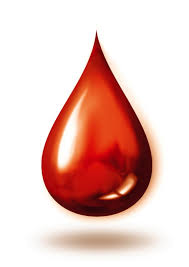3.1 Intro To Matter

5 Questions | Total Attempts: 61SettingsMatter is a very important, seeing as it is the very thing that chemists study. In this unit we will learn a little bit more about the forms of matter. Watch, read, and answer the questions

• 1.
All matter can be classified as either an element, molecule, or mixture. Water (), for example would be classified as a(n) _________________.
• A.

Element

• B.

Molecule

• C.

Mixture

• D.

None of the above

• 2.
Hydrogen () is is a molecule.
• A.

True

• B.

False

• 3.
NaCl, commonly known as table salt, is a _______________.
• A.

Element

• B.

Molecule

• C.

Mixture

• D.

None of the above

• 4.
This pencil uses graphite, a common form of carbon. Graphite is a(n) _____________.
• A.

Element

• B.

Molecule

• C.

Mixture

• D.

All of the above

• 5.
All matter can be classified as either an element, molecule, or mixture. Blood, for example would be classified as a(n) _________________.
• A.

Element

• B.

Molecule

• C.

Mixture

• D.

None of the above

Related TopicsBack to top